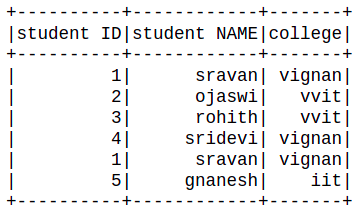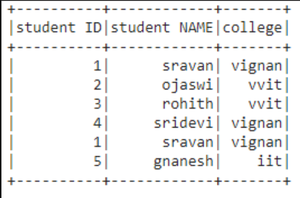Related Articles

# How to select last row and access PySpark dataframe by index ?

• Last Updated : 22 Jun, 2021

In this article, we will discuss how to select the last row and access pyspark dataframe by index.

Creating dataframe for demonstration:

## Python3

 `# importing module``import` `pyspark` `# importing sparksession from pyspark.sql module``from` `pyspark.sql ``import` `SparkSession` `# creating sparksession and giving an app name``spark ``=` `SparkSession.builder.appName(``'sparkdf'``).getOrCreate()` `# list  of students  data``data ``=` `[[``"1"``,``"sravan"``,``"vignan"``],``       ``[``"2"``,``"ojaswi"``,``"vvit"``],``       ``[``"3"``,``"rohith"``,``"vvit"``],``       ``[``"4"``,``"sridevi"``,``"vignan"``],``       ``[``"1"``,``"sravan"``,``"vignan"``],``       ``[``"5"``,``"gnanesh"``,``"iit"``]]` `# specify column names``columns ``=` `[``'student ID'``,``'student NAME'``,``'college'``]` `# creating a dataframe from the lists of data``dataframe ``=` `spark.createDataFrame(data,columns)` `# show dataframe``dataframe.show()`

Output:### Select last row from dataframe

Example 1: Using tail() function.

This function is used to access the last row of the dataframe

Syntax: dataframe.tail(n)

where

1. n is the number of rows to be selected from the last.
2. dataframe is the input dataframe

We can use n = 1 to select only last row.

Example 1: Selecting last row.

## Python3

 `# access last row of the dataframe``dataframe.tail(``1``)`

Output:

[Row(student ID=’5′, student NAME=’gnanesh’, college=’iit’)]

Example 2: Python program to access last N rows.

## Python3

 `# access last 5 rows of the``# dataframe``dataframe.tail(``5``)`

Output:

```[Row(student ID='2', student NAME='ojaswi', college='vvit'),
Row(student ID='3', student NAME='rohith', college='vvit'),
Row(student ID='4', student NAME='sridevi', college='vignan'),
Row(student ID='1', student NAME='sravan', college='vignan'),
Row(student ID='5', student NAME='gnanesh', college='iit')]```

### Access the dataframe by column index

Here we are going to select the dataframe based on the column number. For selecting a specific column by using column number in the pyspark dataframe, we are using select() function

Syntax: dataframe.select(dataframe.columns[column_number]).show()

where,

1. dataframe is the dataframe name
2. dataframe.columns[]: is the method which can take column number as an input and select those column
3. show() function is used to display the selected column

Example 1: Python program to access column based on column number

## Python3

 `# select column with column number 1``dataframe.select(dataframe.columns[``1``]).show()`

Output:

```+------------+
|student NAME|
+------------+
|      sravan|
|      ojaswi|
|      rohith|
|     sridevi|
|      sravan|
|     gnanesh|
+------------+```

Example 2: Accessing multiple columns based on column number, here we are going to select multiple columns by using the slice operator, It can access upto n columns

Syntax: dataframe.select(dataframe.columns[column_start:column_end]).show()

where: column_start is the starting index and column_end is the ending index.

## Python3

 `# select column with column number slice``# operator``dataframe.select(dataframe.columns[``0``:``3``]).show()`

Output:Attention geek! Strengthen your foundations with the Python Programming Foundation Course and learn the basics.

To begin with, your interview preparations Enhance your Data Structures concepts with the Python DS Course. And to begin with your Machine Learning Journey, join the Machine Learning – Basic Level Course

My Personal Notes arrow_drop_up